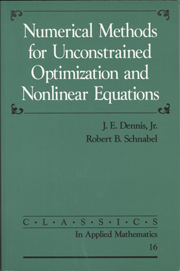Skip to content# Numerical Methods for Unconstrained Optimization and Nonlinear Equations

## £48.99

• Date Published: December 1996
• availability: This item is not supplied by Cambridge University Press in your region. Please contact Soc for Industrial & Applied Mathematics for availability.
• format: Paperback
• isbn: 9780898713640

## £48.99 Paperback

Unavailable

### Looking for an inspection copy?

This title is not currently available on inspection

Description
Product filter button
Description
Contents
Resources
Courses
• This book has become the standard for a complete, state-of-the-art description of the methods for unconstrained optimization and systems of nonlinear equations. Originally published in 1983, it provides information needed to understand both the theory and the practice of these methods and provides pseudocode for the problems. The algorithms covered are all based on Newton's method or 'quasi-Newton' methods, and the heart of the book is the material on computational methods for multidimensional unconstrained optimization and nonlinear equation problems. The republication of this book by SIAM is driven by a continuing demand for specific and sound advice on how to solve real problems.

## Customer reviews

### Not yet reviewed

Be the first to review

Review was not posted due to profanity

×

## , create a review

(If you're not , sign out)

Your review must be a minimum of 12 words.

×

## Product details

• Date Published: December 1996
• format: Paperback
• isbn: 9780898713640
• length: 394 pages
• dimensions: 233 x 150 x 23 mm
• weight: 0.522kg
• availability: This item is not supplied by Cambridge University Press in your region. Please contact Soc for Industrial & Applied Mathematics for availability.

Preface
1. Introduction. Problems to be considered
Characteristics of 'real-world' problems
Finite-precision arithmetic and measurement of error
Exercises
2. Nonlinear Problems in One Variable. What is not possible
Newton's method for solving one equation in one unknown
Convergence of sequences of real numbers
Convergence of Newton's method
Globally convergent methods for solving one equation in one uknown
Methods when derivatives are unavailable
Minimization of a function of one variable
Exercises
3. Numerical Linear Algebra Background. Vector and matrix norms and orthogonality
Solving systems of linear equations—matrix factorizations
Errors in solving linear systems
Updating matrix factorizations
Eigenvalues and positive definiteness
Linear least squares
Exercises
4. Multivariable Calculus Background
Derivatives and multivariable models
Multivariable finite-difference derivatives
Necessary and sufficient conditions for unconstrained minimization
Exercises
5. Newton's Method for Nonlinear Equations and Unconstrained Minimization. Newton's method for systems of nonlinear equations
Local convergence of Newton's method
The Kantorovich and contractive mapping theorems
Finite-difference derivative methods for systems of nonlinear equations
Newton's method for unconstrained minimization
Finite difference derivative methods for unconstrained minimization
Exercises
6. Globally Convergent Modifications of Newton's Method. The quasi-Newton framework
Descent directions
Line searches
The model-trust region approach
Global methods for systems of nonlinear equations
Exercises
7. Stopping, Scaling, and Testing. Scaling
Stopping criteria
Testing
Exercises
8. Secant Methods for Systems of Nonlinear Equations. Broyden's method
Local convergence analysis of Broyden's method
Implementation of quasi-Newton algorithms using Broyden's update
Other secant updates for nonlinear equations
Exercises
9. Secant Methods for Unconstrained Minimization. The symmetric secant update of Powell
Local convergence of positive definite secant methods
Implementation of quasi-Newton algorithms using the positive definite secant update
Another convergence result for the positive definite secant method
Other secant updates for unconstrained minimization
Exercises
10. Nonlinear Least Squares. The nonlinear least-squares problem
Gauss-Newton-type methods
Full Newton-type methods
Other considerations in solving nonlinear least-squares problems
Exercises
11. Methods for Problems with Special Structure. The sparse finite-difference Newton method
Sparse secant methods
Analyzing least-change secant methods
Exercises
Appendix A. A Modular System of Algorithms for Unconstrained Minimization and Nonlinear Equations (by Robert Schnabel)
Appendix B. Test Problems (by Robert Schnabel)
References
Author Index
Subject Index.

• ## Authors

J. E. Dennis

Robert B. Schnabel

Not already registered? Create an account now. ×

### Sorry, this resource is locked

» Proceed

You are now leaving the Cambridge University Press website. Your eBook purchase and download will be completed by our partner www.ebooks.com. Please see the permission section of the www.ebooks.com catalogue page for details of the print & copy limits on our eBooks.

×